×#### Thank you for registering.

One of our academic counsellors will contact you within 1 working day.

Click to Chat

1800-1023-196

+91-120-4616500

CART 0

• 0

MY CART (5)

Use Coupon: CART20 and get 20% off on all online Study Material

ITEM
DETAILS
MRP
DISCOUNT
FINAL PRICE
Total Price: Rs.

There are no items in this cart.
Continue Shopping• Complete JEE Main/Advanced Course and Test Series
• OFFERED PRICE: Rs. 15,900
• View Details

```Chapter 1: Rational Numbers Exercise – 1.6

Question: 1

Verify the property x × y = y × x by taking:Solution:Question: 2

Verify the property: x × (y × z) = (x × y) × z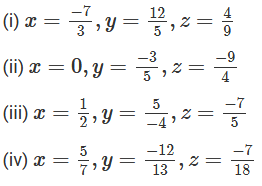Solution:

We have to verify that, x × (y × z) = (x × y) × z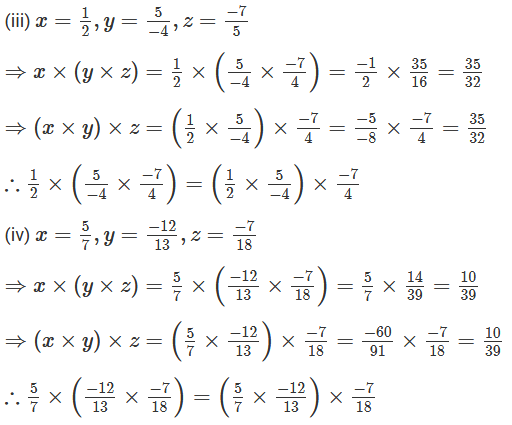Question: 3

Verify the property:  x × (y × z) = x × y + x × z: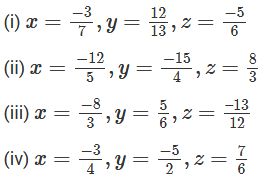Solution:

We have to verify that, x × (y × z) = x × y + x × z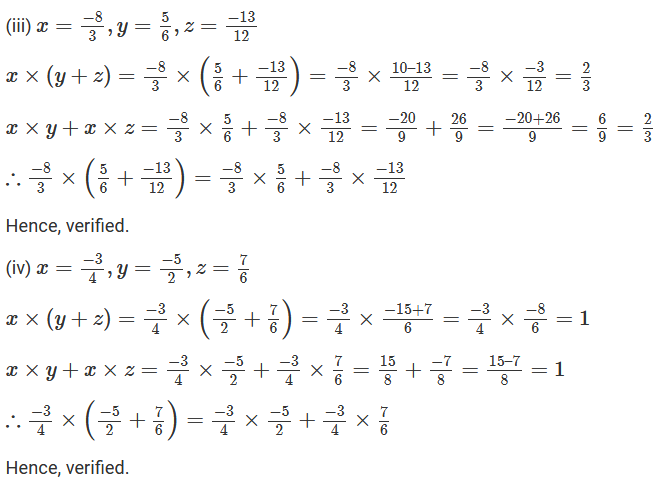Question: 4

Use the distributivity of multiplication of rational numbers over their addition to simplify: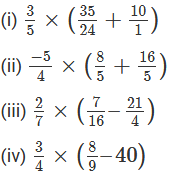Solution: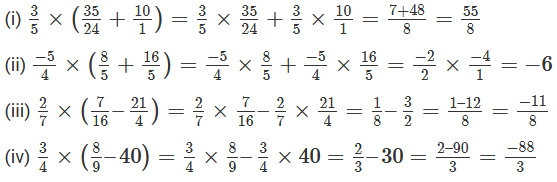Question: 5

Find the multiplicative inverse (reciprocal) of each of the following rational numbers:Solution:Question: 6

Name the property of multiplication of rational numbers illustrated by the following statements: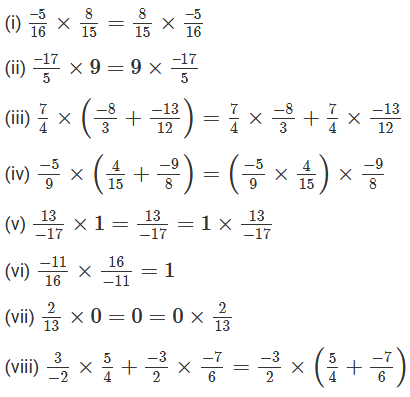Solution:

(i) Commutative property

(ii) Commutative Property

(iii) Distributivity of multiplication over addition

(iv) Associativity of multiplication.

(v) The existence of identity for multiplication.

(vi) Existence of multiplicative inverse

(vii) Multiplication by 0

(viii) Distributive property

Question: 7

Fill in the blanks:

(i) The product of two positive rational numbers is always ……………….

(ii) The product of a positive rational number and a negative rational number is always ……………

(iii) The product of two negative rational numbers is always …………………

(iv) The reciprocal of a positive rational number is ……………….

(v) The reciprocal of a negative rational number is ……………….

(vi) Zero has ………… reciprocal.

(vii) The product of a rational number and its reciprocal is ……………….

(viii)  The numbers ………. and ……….. are their own reciprocals.

(ix) If a is reciprocal of b, then the reciprocal of b is …………………

(x) The number 0 is …………. The reciprocal of any number.

(xi) Reciprocal of 1a, a ≠ 0 is …………………

(xii) (17 × 12)-1 = (17)-1 ×………

Solution:

(i) Positive

(ii) Negative

(iii) Positive

(iv) Positive

(v) Negative

(vi) No

(vii) 1

(viii) -1 and 1

(ix) a

(x) not

(xi) a

(xii) 12−1

Question: 8

Fill in the blanks: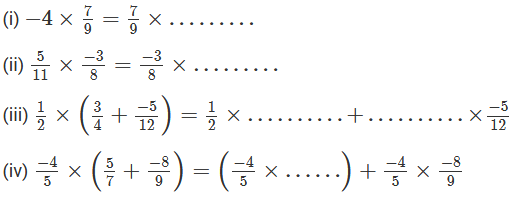Solution:```### Course Features

• 728 Video Lectures
• Revision Notes
• Previous Year Papers
• Mind Map
• Study Planner
• NCERT Solutions
• Discussion Forum
• Test paper with Video Solution